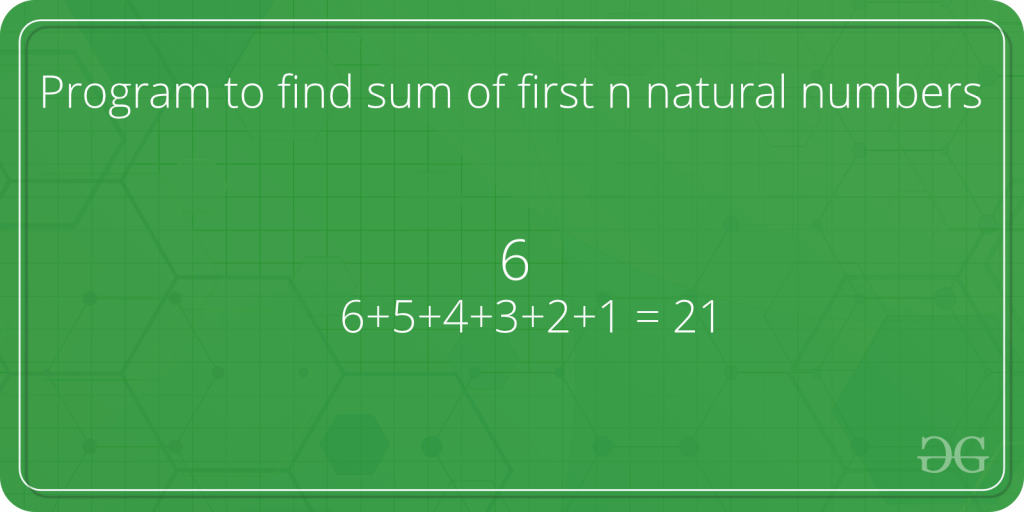# Sum of natural numbers using recursion

• Difficulty Level : Easy
• Last Updated : 07 Apr, 2021Given a number n, find sum of first n natural numbers. To calculate the sum, we will use a recursive function recur_sum().
Examples :

Attention reader! All those who say programming isn't for kids, just haven't met the right mentors yet. Join the  Demo Class for First Step to Coding Coursespecifically designed for students of class 8 to 12.

The students will get to learn more about the world of programming in these free classes which will definitely help them in making a wise career choice in the future.

```Input : 3
Output : 6
Explanation : 1 + 2 + 3 = 6

Input : 5
Output : 15
Explanation : 1 + 2 + 3 + 4 + 5 = 15```

Below is code to find the sum of natural numbers up to n using recursion :

## C++

 `// C++ program to find the``// sum of natural numbers up``// to n using recursion``#include ``using` `namespace` `std;` `// Returns sum of first``// n natural numbers``int` `recurSum(``int` `n)``{``    ``if` `(n <= 1)``        ``return` `n;``    ``return` `n + recurSum(n - 1);``}` `// Driver code``int` `main()``{``    ``int` `n = 5;``    ``cout << recurSum(n);``    ``return` `0;``}`

## Java

 `// Java program to find the``// sum of natural numbers up``// to n using recursion``import` `java.util.*;``import` `java.lang.*;` `class` `GFG``{` `    ``// Returns sum of first``    ``// n natural numbers``    ``public` `static` `int` `recurSum(``int` `n)``    ``{``        ``if` `(n <= ``1``)``            ``return` `n;``        ``return` `n + recurSum(n - ``1``);``    ``}``    ` `    ``// Driver code``    ``public` `static` `void` `main(String args[])``    ``{``        ``int` `n = ``5``;``        ``System.out.println(recurSum(n));``    ``}``}` `// This code is contributed by Sachin Bisht`

## Python

 `# Python code to find sum``# of natural numbers upto``# n using recursion` `# Returns sum of first``# n natural numbers``def` `recurSum(n):``    ``if` `n <``=` `1``:``        ``return` `n``    ``return` `n ``+` `recurSum(n ``-` `1``)` `# Driver code``n ``=` `5``print``(recurSum(n))`

## C#

 `// C# program to find the``// sum of natural numbers``// up to n using recursion``using` `System;` `class` `GFG``{` `    ``// Returns sum of first``    ``// n natural numbers``    ``public` `static` `int` `recurSum(``int` `n)``    ``{``        ``if` `(n <= 1)``            ``return` `n;``        ``return` `n + recurSum(n - 1);``    ``}``    ` `    ``// Driver code``    ``public` `static` `void` `Main()``    ``{``        ``int` `n = 5;``        ``Console.WriteLine(recurSum(n));``    ``}``}` `// This code is contributed by vt_m`

## PHP

 ``

## Javascript

 ``

Output :

`15 `

My Personal Notes arrow_drop_up